true
true

Meenakshi A.

Airoli, Mumbai, India - 400701# Meenakshi A.

## Tutor

Airoli, Mumbai, India - 400701.

3.4

UrbanPro Rating

Details verified of Meenakshi A.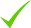IdentityEducation

Know how UrbanPro verifies Tutor details

Identity is verified based on matching the details uploaded by the Tutor with government databases.

## Overview

I can teach each and every topics very easily and make it stronger for the students.

English

Hindi

## Education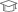Jnvu 2010

Bachelor of Education (B.Ed.)Symbiosis 2015Jnvu 2017

Master of Commerce (M.Com.)

Airoli, Mumbai, India - 400701

## Verified Info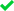Phone VerifiedEmail Verified

Report this Profile

Is this listing inaccurate or duplicate? Any other problem?

Type the letters as shown below *

Please enter the letters as show below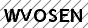Class 11 Tuition

Class Location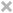Student's HomeTutor's HomeOnline (video chat via skype, google hangout etc)

Years of Experience in Class 11 Tuition

2

Board

State

Taught in School or College

Yes

## Reviews

this is test message this is test message this is test message this is test message this is test message this is test message this is test message

No Reviews yet! Be the first one to Review

## FAQs

1. Which school boards of Class 12 do you teach for?

State

2. Have you ever taught in any School or College?

Yes

3. Which classes do you teach?

I teach Class 10 Tuition, Class 11 Tuition, Class 12 Tuition, Class 6 Tuition, Class 7 Tuition, Class 8 Tuition, Class 9 Tuition and Class I-V Tuition Classes.

4. Do you provide a demo class?

No, I don't provide a demo class.

5. How many years of experience do you have?

I have been teaching for 2 years.

## Answers by Meenakshi A. (6)

Answered on 15/09/2019 Tuition/Class VI-VIII Tuition Tuition/Class VI-VIII Tuition/Mathematics

If 1 2/5 is mixed fraction Then solution will be 1 2/5=7/5. ,4/15 L.c.m=15 21/15+4/15=25/15 5/3 If it is 12/5 Then 12/5+4/15 Lcm same 15 36/15+4/15=40/15 8/3
Dislike Bookmark

Answered on 15/09/2019 Tuition/Class VI-VIII Tuition Tuition/Class VI-VIII Tuition/Mathematics

The question is not clear...1 2/5 is in mixed fraction...or it is 12 /5... If it is 1 2/5(mixed fraction) Solution will be like this... 1 2/5=7/5 2nd fraction is 4/15 L.c.m =15.... 21/15 + 4/15=25/15answer If the first fraction is 5/3 Solution will like this... L.c.m =15 36/15 + 4/15=40/15 ... ...more

The question is not clear...1 2/5 is in mixed fraction...or it is 12 /5...

If it is 1 2/5(mixed fraction)

Solution will be like this...

1 2/5=7/5

2nd fraction is 4/15

L.c.m =15....

If the first fraction is 5/3

Solution will like this...

L.c.m =15

36/15 + 4/15=40/15

8/3

Dislike Bookmark

Answered on 15/09/2019 Tuition/Class VI-VIII Tuition Tuition/Class VI-VIII Tuition/Mathematics

The question is not clear...1 2/5 is in mixed fraction...or it is 12 /5... If it is 1 2/5(mixed fraction) Solution will be like this... 1 2/5=7/5 2nd fraction is 4/15 L.c.m =15.... 21/15 + 4/15=25/15answer If the first fraction is 5/3 Solution will like this... L.c.m =15 36/15 + 4/15=40/15 ... ...more

The question is not clear...1 2/5 is in mixed fraction...or it is 12 /5...

If it is 1 2/5(mixed fraction)

Solution will be like this...

1 2/5=7/5

2nd fraction is 4/15

L.c.m =15....

If the first fraction is 5/3

Solution will like this...

L.c.m =15

36/15 + 4/15=40/15

8/3

Dislike Bookmark

Answered on 15/09/2019 Tuition/Class VI-VIII Tuition Tuition/Class VI-VIII Tuition/Mathematics

The question is not clear...1 2/5 is in mixed fraction...or it is 12 /5... If it is 1 2/5(mixed fraction) Solution will be like this... 1 2/5=7/5 2nd fraction is 4/15 L.c.m =15.... 21/15 + 4/15=25/15answer If the first fraction is 5/3 Solution will like this... L.c.m =15 36/15 + 4/15=40/15 ... ...more

The question is not clear...1 2/5 is in mixed fraction...or it is 12 /5...

If it is 1 2/5(mixed fraction)

Solution will be like this...

1 2/5=7/5

2nd fraction is 4/15

L.c.m =15....

If the first fraction is 5/3

Solution will like this...

L.c.m =15

36/15 + 4/15=40/15

8/3

Dislike Bookmark

Answered on 15/09/2019 Tuition/Class VI-VIII Tuition Tuition/Class VI-VIII Tuition/Mathematics

The question is not clear...1 2/5 is in mixed fraction...or it is 12 /5... If it is 1 2/5(mixed fraction) Solution will be like this... 1 2/5=7/5 2nd fraction is 4/15 L.c.m =15.... 21/15 + 4/15=25/15answer If the first fraction is 5/3 Solution will like this... L.c.m =15 36/15 + 4/15=40/15 ... ...more

The question is not clear...1 2/5 is in mixed fraction...or it is 12 /5...

If it is 1 2/5(mixed fraction)

Solution will be like this...

1 2/5=7/5

2nd fraction is 4/15

L.c.m =15....

If the first fraction is 5/3

Solution will like this...

L.c.m =15

36/15 + 4/15=40/15

8/3

Dislike Bookmark
Class 11 Tuition 3.4

Class LocationStudent's HomeTutor's HomeOnline (video chat via skype, google hangout etc)

Years of Experience in Class 11 Tuition

2

Board

State

Taught in School or College

Yes

Class 12 Tuition 3.4

Class LocationStudent's HomeTutor's HomeOnline (video chat via skype, google hangout etc)

Years of Experience in Class 12 Tuition

2

Board

State

Taught in School or College

Yes

Class 6 Tuition 3.4

Class LocationStudent's HomeTutor's HomeOnline (video chat via skype, google hangout etc)

Years of Experience in Class 6 Tuition

10

Board

CBSE, ICSE, State

CBSE Subjects taught

Hindi, Science, Mathematics, Social Science, EVS

ICSE Subjects taught

Mathematics, Hindi, EVS

Taught in School or College

Yes

State Syllabus Subjects taught

Social science, Hindi, Mathematics, EVS, English, Science

Class 7 Tuition 3.4

Class LocationStudent's HomeTutor's HomeOnline (video chat via skype, google hangout etc)

Years of Experience in Class 7 Tuition

10

Board

CBSE, ICSE, State

CBSE Subjects taught

Hindi, Science, Mathematics, Social Science, EVS

ICSE Subjects taught

Mathematics, Hindi, EVS

Taught in School or College

Yes

State Syllabus Subjects taught

Social science, Hindi, Mathematics, EVS, English, Science

Class 8 Tuition 3.4

Class LocationStudent's HomeTutor's HomeOnline (video chat via skype, google hangout etc)

Years of Experience in Class 8 Tuition

10

Board

CBSE, ICSE, State

CBSE Subjects taught

Hindi, Science, Mathematics, Social Science, EVS

ICSE Subjects taught

Mathematics, Hindi, EVS

Taught in School or College

Yes

State Syllabus Subjects taught

Social science, Hindi, Mathematics, EVS, English, Science

Class 9 Tuition 3.4

Class LocationStudent's HomeTutor's HomeOnline (video chat via skype, google hangout etc)

Years of Experience in Class 9 Tuition

10

Board

CBSE, State

CBSE Subjects taught

Social science, Hindi, Science, Accountancy, English, Mathematics

Taught in School or College

Yes

State Syllabus Subjects taught

EVS, Science, Social Science, Hindi, English, Mathematics

Class 10 Tuition 3.4

Class LocationStudent's HomeTutor's HomeOnline (video chat via skype, google hangout etc)

Years of Experience in Class 10 Tuition

10

Board

CBSE, State

CBSE Subjects taught

Social science, Hindi, Science, Accountancy, English, Mathematics

Taught in School or College

Yes

State Syllabus Subjects taught

EVS, Science, Social Science, Hindi, English, Mathematics

Class I-V Tuition 3.4

Class LocationStudent's HomeTutor's HomeOnline (video chat via skype, google hangout etc)

Years of Experience in Class I-V Tuition

10

Board

CBSE, State, ICSE

CBSE Subjects taught

English, EVS, Science, Mathematics, Social Science, Hindi, Computers

ICSE Subjects taught

Science, Computer science, English, Mathematics, Hindi, Social Studies, EVS

Taught in School or College

Yes

State Syllabus Subjects taught

Social Science, Mathematics, EVS, Hindi, Science, English

this is test message this is test message this is test message this is test message this is test message this is test message this is test message

No Reviews yet! Be the first one to Review

## Answers by Meenakshi A. (6)

Answered on 15/09/2019 Tuition/Class VI-VIII Tuition Tuition/Class VI-VIII Tuition/Mathematics

If 1 2/5 is mixed fraction Then solution will be 1 2/5=7/5. ,4/15 L.c.m=15 21/15+4/15=25/15 5/3 If it is 12/5 Then 12/5+4/15 Lcm same 15 36/15+4/15=40/15 8/3
Dislike Bookmark

Answered on 15/09/2019 Tuition/Class VI-VIII Tuition Tuition/Class VI-VIII Tuition/Mathematics

The question is not clear...1 2/5 is in mixed fraction...or it is 12 /5... If it is 1 2/5(mixed fraction) Solution will be like this... 1 2/5=7/5 2nd fraction is 4/15 L.c.m =15.... 21/15 + 4/15=25/15answer If the first fraction is 5/3 Solution will like this... L.c.m =15 36/15 + 4/15=40/15 ... ...more

The question is not clear...1 2/5 is in mixed fraction...or it is 12 /5...

If it is 1 2/5(mixed fraction)

Solution will be like this...

1 2/5=7/5

2nd fraction is 4/15

L.c.m =15....

If the first fraction is 5/3

Solution will like this...

L.c.m =15

36/15 + 4/15=40/15

8/3

Dislike Bookmark

Answered on 15/09/2019 Tuition/Class VI-VIII Tuition Tuition/Class VI-VIII Tuition/Mathematics

The question is not clear...1 2/5 is in mixed fraction...or it is 12 /5... If it is 1 2/5(mixed fraction) Solution will be like this... 1 2/5=7/5 2nd fraction is 4/15 L.c.m =15.... 21/15 + 4/15=25/15answer If the first fraction is 5/3 Solution will like this... L.c.m =15 36/15 + 4/15=40/15 ... ...more

The question is not clear...1 2/5 is in mixed fraction...or it is 12 /5...

If it is 1 2/5(mixed fraction)

Solution will be like this...

1 2/5=7/5

2nd fraction is 4/15

L.c.m =15....

If the first fraction is 5/3

Solution will like this...

L.c.m =15

36/15 + 4/15=40/15

8/3

Dislike Bookmark

Answered on 15/09/2019 Tuition/Class VI-VIII Tuition Tuition/Class VI-VIII Tuition/Mathematics

The question is not clear...1 2/5 is in mixed fraction...or it is 12 /5... If it is 1 2/5(mixed fraction) Solution will be like this... 1 2/5=7/5 2nd fraction is 4/15 L.c.m =15.... 21/15 + 4/15=25/15answer If the first fraction is 5/3 Solution will like this... L.c.m =15 36/15 + 4/15=40/15 ... ...more

The question is not clear...1 2/5 is in mixed fraction...or it is 12 /5...

If it is 1 2/5(mixed fraction)

Solution will be like this...

1 2/5=7/5

2nd fraction is 4/15

L.c.m =15....

If the first fraction is 5/3

Solution will like this...

L.c.m =15

36/15 + 4/15=40/15

8/3

Dislike Bookmark

Answered on 15/09/2019 Tuition/Class VI-VIII Tuition Tuition/Class VI-VIII Tuition/Mathematics

The question is not clear...1 2/5 is in mixed fraction...or it is 12 /5... If it is 1 2/5(mixed fraction) Solution will be like this... 1 2/5=7/5 2nd fraction is 4/15 L.c.m =15.... 21/15 + 4/15=25/15answer If the first fraction is 5/3 Solution will like this... L.c.m =15 36/15 + 4/15=40/15 ... ...more

The question is not clear...1 2/5 is in mixed fraction...or it is 12 /5...

If it is 1 2/5(mixed fraction)

Solution will be like this...

1 2/5=7/5

2nd fraction is 4/15

L.c.m =15....

If the first fraction is 5/3

Solution will like this...

L.c.m =15

36/15 + 4/15=40/15

8/3

Dislike Bookmark

Meenakshi A. describes herself as Tutor. She conducts classes in Class 10 Tuition, Class 11 Tuition and Class 12 Tuition. Meenakshi is located in Airoli, Mumbai. Meenakshi takes Regular Classes- at her Home. She has 10 years of teaching experience . Meenakshi has completed Bachelor of Education (B.Ed.) from Jnvu in 2010, Master of Business Administration (M.B.A.) from Symbiosis in 2015 and Master of Commerce (M.Com.) from Jnvu in 2017. She is well versed in English and Hindi.

•• Want to learn from Meenakshi A.?

• Contact Now
X

Recommended Profiles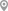Airoli, MumbaiAiroli, MumbaiAiroli, MumbaiAiroli, MumbaiAiroli, MumbaiAiroli, Mumbai

X

Let us shortlist and give the best tutors and institutes.

or

Send Enquiry to Meenakshi A.

Let Meenakshi A. know you are interested in their class